• ## Hyperopt 参数优化

万次阅读 多人点赞 2018-08-28 20:02:03
Hyperopt（Hyper-parameter Optimization）用于模型选择和参数优化参数选择在训练模型时是一个很关键的部分。然而存在这样的问题，一方面参数选择背后包含着一定的数学原理，对于新手来说难上手；另一方面，一个...
翻译自https://districtdatalabs.silvrback.com/parameter-tuning-with-hyperopt
Parameter Tuning with Hyperopt –Kris Wright

概述

Hyperopt可以帮助快速进行机器学习模型参数调试。通常情况下有两种类型的参数调试方法，网格搜索（grid search）和随机搜索（random search）。网格搜索速度慢但是适用于需要随整个参数空间进行搜索的情况；随机搜索速度很快但是容易遗漏一些重要信息。幸运的是，我们有第三个选择：贝叶斯优化（Bayesian optimization）。这里我们关注贝叶斯优化在Python中的实现：Hyperopt模块。

使用贝叶斯优化进行参数调试允许我们获得给定模型的最优参数，如，逻辑回归（ logistic regression），因此我们可以进行最优模型选择。通常情况下，机器学习工程师或数据科学家会对一些模型进行一些形式的手动参数调试（如网格搜索或随机搜索），如决策树（decision tree）、支持向量机（support vector machine）、k最近邻（k nearest neighbors）-然后比较准确度得分（accuracy score）进而选择性能表现最好的一组参数给模型使用。这种方法可能会产生次优模型（sub-optimal models）。数据科学家可能会给决策树选择一组最优参数，但是对SVM却不是最优的，这就意味着模型比较是有缺陷的。KNN每次都可以打败SVM，如果SVM的参数选择很差的话。贝叶斯优化允许数据科学家找到所有模型的最优参数，然后比较所有使用了最佳参数的模型。这样的话，在模型选择中，就可以比较最优的决策树和最优的支持向量机。只有这样才能保证我们选择和使用了实际最好的模型。

本文涉及的主题包括：
- 评估函数（Objective functions）
- 搜索空间（Search spaces）
- 存储评价试验（Storing evaluation trials）
- 可视化（Visualization）
- 鸢尾花数据集实例（Full example on a classic dataset: Iris）

目标函数（Objective Functions - A Motivating Example）

假设有一个定义在摸个范围内的函数，你想要使这个函数最小化，也就是说，你想要找到一个输入值，使得函数的输出结果是最小的。如下面的例子，找到一个x，使得线性函数y（x）=x取得最小值。

from hyperopt import fmin, tpe, hp
best = fmin(
fn=lambda x: x,
space=hp.uniform('x', 0, 1),
algo=tpe.suggest,
max_evals=100)
print best

下面进行分解说明。
函数fmin首先要接收一个函数fn来最小化，这里我们指定fn为一个匿名函数lambda x: x，实际上这个函数可以是任何有效的带有返回值的函数（valid value-returning function），如回归中的平均绝对误差（mean absolute error）。
第二个参数space指定了搜索空间（search space），在这个例子中为在0到1范围之间的数字。hp.uniform是一个内置的（ built-in）hyperopt函数，包括三个参数：name：x，范围的上界和下届：0和1。
参数algo接收一个搜索算法（serach algorithm），这个例子中tpe代表tree of Parzen estimators。这个话题超出了这个博客文章的范围，更多关于tpe的内容请参考这里。algo参数也可以设置成hyperopt.random，但我们在此不做介绍，因为这是众所周知的搜索策略。

最后，我们指定fmin函数执行的最大次数max_evals。fmin函数返回一个python字典。函数输出的一个例子：{‘x’: 0.000269455723739237}。
下面是一张函数图像，红色的点是我们要找的x的位置。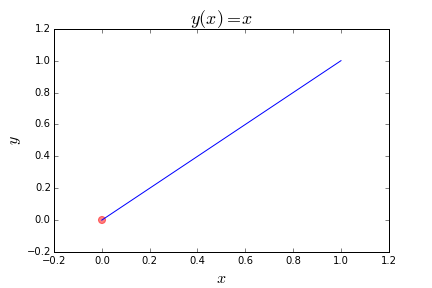另一个例子（More Complicated Examples）

这里有个更复杂的目标函数：lambda x: (x-1)**2，这次我们试着最小化一个二次方程y(x)=(x−1)2$y\left(x\right)=\left(x-1{\right)}^{2}$$y(x) = (x-1)^2$。因此，我们将搜索空间更改为包括我们已经知道的最优值x=1以及加上两侧的一些次优范围：hp.uniform(‘x’, -2, 2)。
我们可以得到：

best = fmin(
fn=lambda x: (x-1)**2,
space=hp.uniform('x', -2, 2),
algo=tpe.suggest,
max_evals=100)
print best

输出为：

{'x': 0.997369045274755}

函数如下图：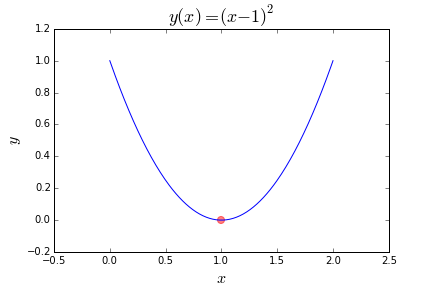除了最小化目标函数，也许我们想使函数值最大化。这种情况下我们只需要返回函数的负值，如函数y(x) = -(x**2)：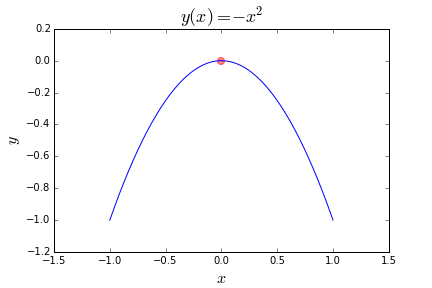如何解决这个问题呢？我们只需要将目标函数改为lambda x: (x**2），求y(x) = -(x**2)得最小值即为求y(x) = -(x**2)的最大值。
同理对于刚开始的线性函数的例子，我们将求最小化改为求最大化，将将目标函数改为lambda x: -x即可。
下面是一个有用许多（给定无穷范围的无穷多个）局部最小值得函数，也可以求最大值。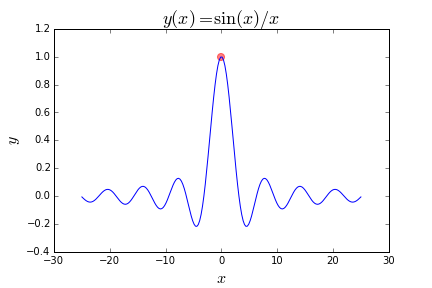搜索空间（Search spaces）

Hyperopt模块包含了一些方便的函数（handy functions）来指定输入参数的范围。我们已经见过hp.uniform。最初，这些是随机搜索空间，但是随着hyperopt的学习（随着从目标函数获得更多的feedback），它会对初始搜索空间的不同部分进行调整和采样，并认为这些部分会给它提供最有意义的反馈。
以下函数将在本文使用：

hp.choice(label, options) ，其中options为 python list 或tuple
hp.normal(label, mu, sigma) ，其中mu 和 sigma 分别为均值和标准差
hp.uniform(label, low, high)，其中low和 high分别为范围的上界和下界

其他还有hp.normal, hp.lognormal, hp.quniform，因为本文不会使用就没有写，可自行了解。
下面定义搜索空间：

import hyperopt.pyll.stochastic

space = {
'x': hp.uniform('x', 0, 1),
'y': hp.normal('y', 0, 1),
'name': hp.choice('name', ['alice', 'bob']),
}

print hyperopt.pyll.stochastic.sample(space)

例子输出：

{'y': -1.4012610048810574, 'x': 0.7258615424906184, 'name': 'alice'}

获取信息和试验（Capturing Info with Trials）

如果能看到hyperopt黑盒里到底发生了什么，那就太好了。Trials对象允许我们这样做。我们只需要再导入几个项目

from hyperopt import fmin, tpe, hp, STATUS_OK, Trials

fspace = {
'x': hp.uniform('x', -5, 5)
}

def f(params):
x = params['x']
val = x**2
return {'loss': val, 'status': STATUS_OK}

trials = Trials()
best = fmin(fn=f, space=fspace, algo=tpe.suggest, max_evals=50, trials=trials)

print 'best:', best

print 'trials:'
for trial in trials.trials[:2]:
print trial

STATUS_OK 和 Trials是新导入的模块，Trials允许我们存储每一时间步长（time step）所存储的信息。然后我们可以输出这些函数在给定时间步长上对给定参数的求值。
输出：

best: {'x': 0.014420181637303776}
trials:
{'refresh_time': None, 'book_time': None, 'misc': {'tid': 0, 'idxs': {'x': }, 'cmd': ('domain_attachment', 'FMinIter_Domain'), 'vals': {'x': [1.9646918559786162]}, 'workdir': None}, 'state': 2, 'tid': 0, 'exp_key': None, 'version': 0, 'result': {'status': 'ok', 'loss': 3.8600140889486996}, 'owner': None, 'spec': None}
{'refresh_time': None, 'book_time': None, 'misc': {'tid': 1, 'idxs': {'x': }, 'cmd': ('domain_attachment', 'FMinIter_Domain'), 'vals': {'x': [-3.9393509404526728]}, 'workdir': None}, 'state': 2, 'tid': 1, 'exp_key': None, 'version': 0, 'result': {'status': 'ok', 'loss': 15.518485832045357}, 'owner': None, 'spec': None}

Trials对象将数据存储为BSON对象，类似于JSON对象一样。BSON来自pymongo模块，着这里我们不讨论细节，但是对于hyperopt有一些高级选项需要使用MongoDB进行分布式计算，因此导入pymongo。
回到上面的输出，’tid’表示时间id，也就是时间步长，范围0到max_evals-1，每次迭代加1；’x’在’vals’键中，也就是每次迭代参数存储的地方；’loss’在’result’键中，是每次迭代目标函数的值。

可视化（Visualization）

这里介绍两种类型可视化，val vs. time和loss vs. val。
首先val vs. time。下面是trials.trials数据描述可视化的代码和样本输出。

f, ax = plt.subplots(1)
xs = [t['tid'] for t in trials.trials]
ys = [t['misc']['vals']['x'] for t in trials.trials]
ax.set_xlim(xs-10, xs[-1]+10)
ax.scatter(xs, ys, s=20, linewidth=0.01, alpha=0.75)
ax.set_title('$x$ $vs$ $t$ ', fontsize=18)
ax.set_xlabel('$t$', fontsize=16)
ax.set_ylabel('$x$', fontsize=16)

输出如下（假设我们将max_evals设置为1000）：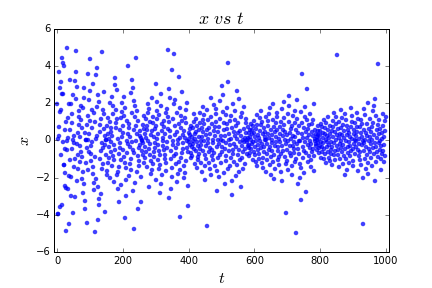我们可以看到，最初算法从整个范围中均匀取值，但是随着时间的增加以及对于参数在目标函数上效果的学习，算法搜索范围越来越集中到最可能取得最优值的范围-0附近。它仍然探索整个解决方案空间，但不太频繁。

loss vs. val的可视化：

f, ax = plt.subplots(1)
xs = [t['misc']['vals']['x'] for t in trials.trials]
ys = [t['result']['loss'] for t in trials.trials]
ax.scatter(xs, ys, s=20, linewidth=0.01, alpha=0.75)
ax.set_title('$val$ $vs$ $x$ ', fontsize=18)
ax.set_xlabel('$x$', fontsize=16)
ax.set_ylabel('$val$', fontsize=16)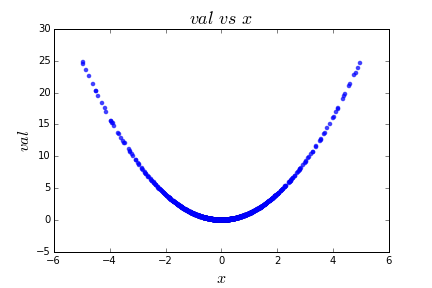这就是我们期望的，因为函数y(x) = x**2是确定性的。
最后，让我们尝试一个更复杂的示例，使用更多的随机性和更多的参数。

鸢尾花数据集（The Iris Dataset）

在本节中，我们将介绍在经典数据集Iris上使用hyperopt进行参数调优的4个完整示例。我们将介绍k近邻(KNN)、支持向量机(SVM)、决策树和随机森林。注意，由于我们试图最大化交叉验证的准确性(下面代码中的acc)，我们必须为hyperopt对这个值进行取负数，因为hyperopt只知道如何最小化函数。最小化函数f等于最大化函数f的复数。
对于这项任务，我们将使用经典的Iris数据集，并进行一些有监督的机器学习。有4个输入特性和3个输出类。这些数据被标记为属于0类、1类或2类，它们映射到不同种类的鸢尾花。输入有4列:萼片长度(sepal length)、萼片宽度(sepal width)、花瓣长度(petal length)和花瓣宽度(pedal width)。输入单位是厘米。我们将使用这4个特性来学习预测三个输出类之一的模型。由于数据是由sklearn提供的，因此它有一个很好的DESCR属性，提供了关于数据集的详细信息。

print iris.feature_names # input names
print iris.target_names # output names
print iris.DESCR # everything else

让我们通过可视化特性和类来更好地了解数据，使用下面的代码。如果还没有安装seaborn，请不要忘记安装pip install seaborn。

import seaborn as sns
sns.set(style="whitegrid", palette="husl")

iris = pd.melt(iris, "species", var_name="measurement")

f, ax = plt.subplots(1, figsize=(15,10))
sns.stripplot(x="measurement", y="value", hue="species", data=iris, jitter=True, edgecolor="white", ax=ax)

如图：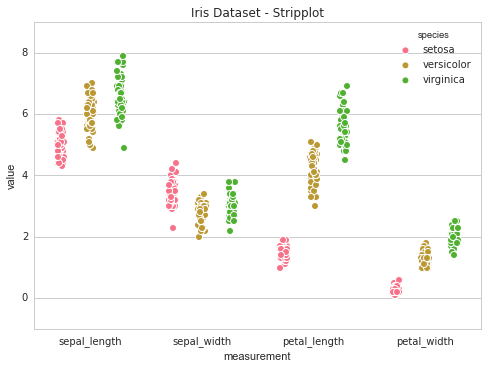K最近邻（K-Nearest Neighbors）

我们现在使用hyperopt找到k近邻(KNN)机器学习模型的最佳参数。KNN模型根据训练数据集中k个最近的数据点的多数类，将测试集中的数据点进行分类。
关于这个算法的更多信息可以在这里找到。下面的代码包含了我们已经讨论过的所有内容。

from sklearn import datasets
X = iris.data
y = iris.target

def hyperopt_train_test(params):
clf = KNeighborsClassifier(**params)
return cross_val_score(clf, X, y).mean()

space4knn = {
'n_neighbors': hp.choice('n_neighbors', range(1,100))
}

def f(params):
acc = hyperopt_train_test(params)
return {'loss': -acc, 'status': STATUS_OK}

trials = Trials()
best = fmin(f, space4knn, algo=tpe.suggest, max_evals=100, trials=trials)
print 'best:'
print best

现在我们来看看输出图。y轴是交叉验证得分，x轴是k-最近邻中的k值。下面是代码及其图像：

f, ax = plt.subplots(1)#, figsize=(10,10))
xs = [t['misc']['vals']['n_neighbors'] for t in trials.trials]
ys = [-t['result']['loss'] for t in trials.trials]
ax.scatter(xs, ys, s=20, linewidth=0.01, alpha=0.5)
ax.set_title('Iris Dataset - KNN', fontsize=18)
ax.set_xlabel('n_neighbors', fontsize=12)
ax.set_ylabel('cross validation accuracy', fontsize=12)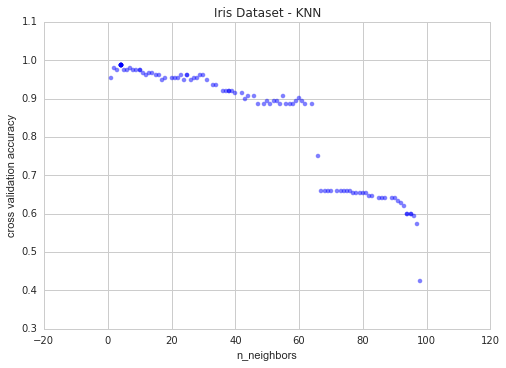当k大于63时，准确率急剧下降。这是由于数据集中每个类的数量。这三个类中的每个类只有50个实例。因此，让我们通过将’n_neighbors’的值限制为较小的值进行深入研究。

from sklearn import datasets
X = iris.data
y = iris.target

def hyperopt_train_test(params):
clf = KNeighborsClassifier(**params)
return cross_val_score(clf, X, y).mean()

space4knn = {
'n_neighbors': hp.choice('n_neighbors', range(1,50))
}

def f(params):
acc = hyperopt_train_test(params)
return {'loss': -acc, 'status': STATUS_OK}

trials = Trials()
best = fmin(f, space4knn, algo=tpe.suggest, max_evals=100, trials=trials)
print 'best:'
print best

下面是当我们运行相同的可视化代码时得到的结果: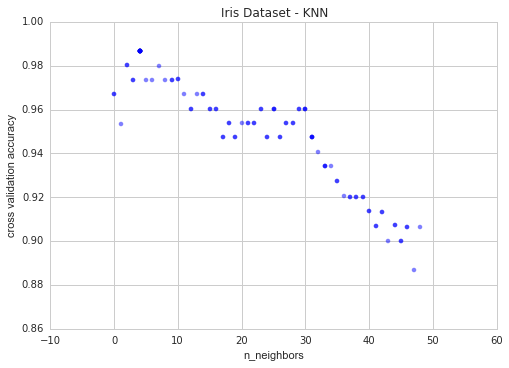现在我们可以清楚地看到k在k = 4处有一个最佳值。

上面的模型不做任何预处理。让我们对我们的特性进行规范化和缩放看看这是否有帮助。使用这段代码:

# now with scaling as an option
from sklearn import datasets
X = iris.data
y = iris.target

def hyperopt_train_test(params):
X_ = X[:]

if 'normalize' in params:
if params['normalize'] == 1:
X_ = normalize(X_)
del params['normalize']

if 'scale' in params:
if params['scale'] == 1:
X_ = scale(X_)
del params['scale']

clf = KNeighborsClassifier(**params)
return cross_val_score(clf, X_, y).mean()

space4knn = {
'n_neighbors': hp.choice('n_neighbors', range(1,50)),
'scale': hp.choice('scale', [0, 1]),
'normalize': hp.choice('normalize', [0, 1])
}

def f(params):
acc = hyperopt_train_test(params)
return {'loss': -acc, 'status': STATUS_OK}

trials = Trials()
best = fmin(f, space4knn, algo=tpe.suggest, max_evals=100, trials=trials)
print 'best:'
print best

像这样画出参数:

parameters = ['n_neighbors', 'scale', 'normalize']
cols = len(parameters)
f, axes = plt.subplots(nrows=1, ncols=cols, figsize=(15,5))
cmap = plt.cm.jet
for i, val in enumerate(parameters):
xs = np.array([t['misc']['vals'][val] for t in trials.trials]).ravel()
ys = [-t['result']['loss'] for t in trials.trials]
xs, ys = zip(\*sorted(zip(xs, ys)))
ys = np.array(ys)
axes[i].scatter(xs, ys, s=20, linewidth=0.01, alpha=0.75, c=cmap(float(i)/len(parameters)))
axes[i].set_title(val)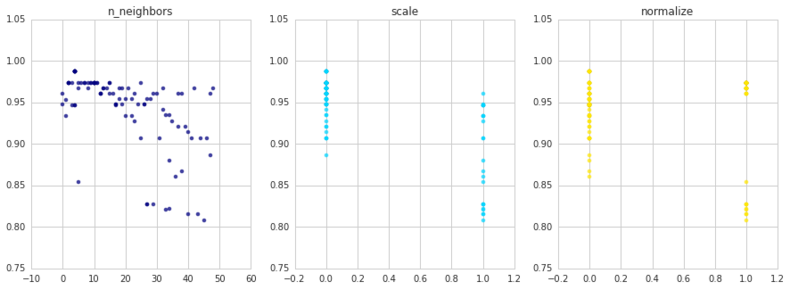我们发现，数据的缩放和/或规范化并不能提高预测的准确性。k的最佳值为4，准确率为98.6%。

这对于一个简单模型KNN的参数调优非常有用。让我们看看支持向量机(SVM)能做些什么。

支持向量机 (SVM)

由于这是一个分类任务，我们将使用sklearn的SVC类。这是代码:

iris = datasets.load_iris()
X = iris.data
y = iris.target

def hyperopt_train_test(params):
X_ = X[:]

if 'normalize' in params:
if params['normalize'] == 1:
X_ = normalize(X_)
del params['normalize']

if 'scale' in params:
if params['scale'] == 1:
X_ = scale(X_)
del params['scale']

clf = SVC(**params)
return cross_val_score(clf, X_, y).mean()

space4svm = {
'C': hp.uniform('C', 0, 20),
'kernel': hp.choice('kernel', ['linear', 'sigmoid', 'poly', 'rbf']),
'gamma': hp.uniform('gamma', 0, 20),
'scale': hp.choice('scale', [0, 1]),
'normalize': hp.choice('normalize', [0, 1])
}

def f(params):
acc = hyperopt_train_test(params)
return {'loss': -acc, 'status': STATUS_OK}

trials = Trials()
best = fmin(f, space4svm, algo=tpe.suggest, max_evals=100, trials=trials)
print 'best:'
print best

parameters = ['C', 'kernel', 'gamma', 'scale', 'normalize']
cols = len(parameters)
f, axes = plt.subplots(nrows=1, ncols=cols, figsize=(20,5))
cmap = plt.cm.jet
for i, val in enumerate(parameters):
xs = np.array([t['misc']['vals'][val] for t in trials.trials]).ravel()
ys = [-t['result']['loss'] for t in trials.trials]
xs, ys = zip(\*sorted(zip(xs, ys)))
axes[i].scatter(xs, ys, s=20, linewidth=0.01, alpha=0.25, c=cmap(float(i)/len(parameters)))
axes[i].set_title(val)
axes[i].set_ylim([0.9, 1.0])

下面是我们得到的: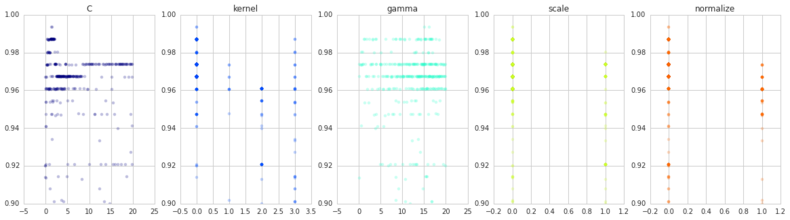同样，缩放和规范化也没有帮助。核函数的首选为最佳(linear)，最佳C值为1.4168540399911616，最佳伽玛值为15.04230279483486。该参数集的分类准确率达到99.3%。

决策树（Decision Trees）

我们将只尝试对决策树的几个参数进行优化。这是代码。

iris = datasets.load_iris()
X_original = iris.data
y_original = iris.target

def hyperopt_train_test(params):
X_ = X[:]
if 'normalize' in params:
if params['normalize'] == 1:
X_ = normalize(X_)
del params['normalize']

if 'scale' in params:
if params['scale'] == 1:
X_ = scale(X_)
del params['scale']
clf = DecisionTreeClassifier(**params)
return cross_val_score(clf, X, y).mean()

space4dt = {
'max_depth': hp.choice('max_depth', range(1,20)),
'max_features': hp.choice('max_features', range(1,5)),
'criterion': hp.choice('criterion', ["gini", "entropy"]),
'scale': hp.choice('scale', [0, 1]),
'normalize': hp.choice('normalize', [0, 1])
}

def f(params):
acc = hyperopt_train_test(params)
return {'loss': -acc, 'status': STATUS_OK}

trials = Trials()
best = fmin(f, space4dt, algo=tpe.suggest, max_evals=300, trials=trials)
print 'best:'
print best

输出如下，准确率为97.3%：

{'max_features': 1, 'normalize': 0, 'scale': 0, 'criterion': 0, 'max_depth': 17}

如图我们可以看到，在不同的尺度值、标准化值和标准值下，性能几乎没有差别。

parameters = ['max_depth', 'max_features', 'criterion', 'scale', 'normalize'] # decision tree
cols = len(parameters)
f, axes = plt.subplots(nrows=1, ncols=cols, figsize=(20,5))
cmap = plt.cm.jet
for i, val in enumerate(parameters):
xs = np.array([t['misc']['vals'][val] for t in trials.trials]).ravel()
ys = [-t['result']['loss'] for t in trials.trials]
xs, ys = zip(\*sorted(zip(xs, ys)))
ys = np.array(ys)
axes[i].scatter(xs, ys, s=20, linewidth=0.01, alpha=0.5, c=cmap(float(i)/len(parameters)))
axes[i].set_title(val)
#axes[i].set_ylim([0.9,1.0])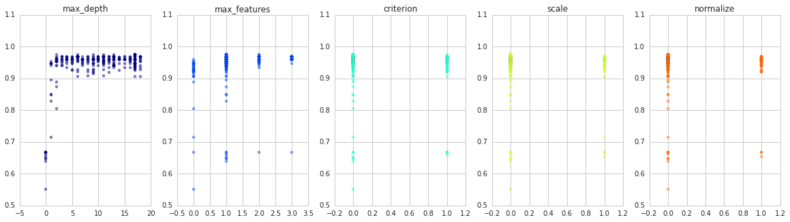随机森林（Random Forests）

让我们看看集成分类器Random Forest发生了什么，它只是一组针对不同大小的数据分区训练的决策树，每个分区对一个输出类进行投票，并选择多数派类作为预测。

iris = datasets.load_iris()
X_original = iris.data
y_original = iris.target

def hyperopt_train_test(params):
X_ = X[:]
if 'normalize' in params:
if params['normalize'] == 1:
X_ = normalize(X_)
del params['normalize']

if 'scale' in params:
if params['scale'] == 1:
X_ = scale(X_)
del params['scale']
clf = RandomForestClassifier(**params)
return cross_val_score(clf, X, y).mean()

space4rf = {
'max_depth': hp.choice('max_depth', range(1,20)),
'max_features': hp.choice('max_features', range(1,5)),
'n_estimators': hp.choice('n_estimators', range(1,20)),
'criterion': hp.choice('criterion', ["gini", "entropy"]),
'scale': hp.choice('scale', [0, 1]),
'normalize': hp.choice('normalize', [0, 1])
}

best = 0
def f(params):
global best
acc = hyperopt_train_test(params)
if acc > best:
best = acc
print 'new best:', best, params
return {'loss': -acc, 'status': STATUS_OK}

trials = Trials()
best = fmin(f, space4rf, algo=tpe.suggest, max_evals=300, trials=trials)
print 'best:'
print best

同样，我们只得到97.3%的准确率，和决策树一样。

下面是绘制参数的代码:

parameters = ['n_estimators', 'max_depth', 'max_features', 'criterion', 'scale', 'normalize']
f, axes = plt.subplots(nrows=2, ncols=3, figsize=(15,10))
cmap = plt.cm.jet
for i, val in enumerate(parameters):
print i, val
xs = np.array([t['misc']['vals'][val] for t in trials.trials]).ravel()
ys = [-t['result']['loss'] for t in trials.trials]
xs, ys = zip(\*sorted(zip(xs, ys)))
ys = np.array(ys)
axes[i/3,i%3].scatter(xs, ys, s=20, linewidth=0.01, alpha=0.5, c=cmap(float(i)/len(parameters)))
axes[i/3,i%3].set_title(val)
#axes[i/3,i%3].set_ylim([0.9,1.0])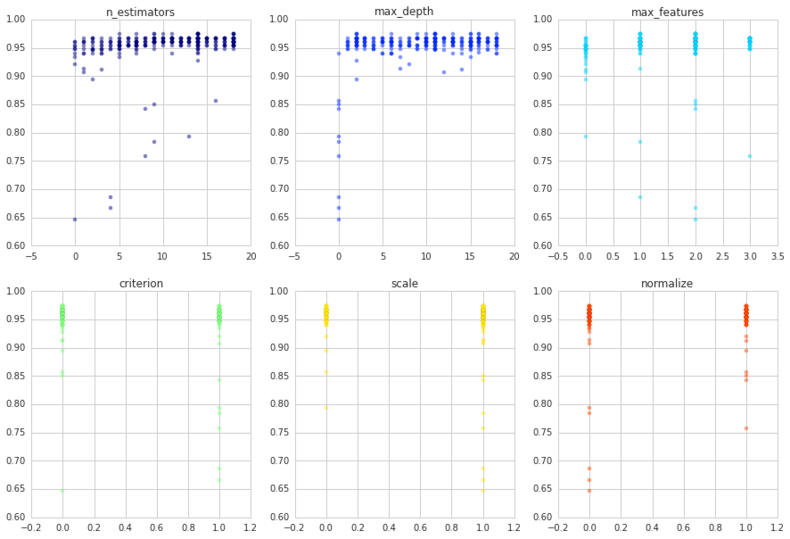所有模型（All Together Now）

虽然自动调优一个模型的参数(例如SVM或KNN)既有趣又有指导意义，但更有用的是一次调优所有的参数并得到一个总体上最好的模型。这使我们能够同时比较所有的参数和所有的模型，这给了我们最好的模型。这是代码。

digits = datasets.load_digits()
X = digits.data
y = digits.target
print X.shape, y.shape

def hyperopt_train_test(params):
t = params['type']
del params['type']
if t == 'naive_bayes':
clf = BernoulliNB(**params)
elif t == 'svm':
clf = SVC(**params)
elif t == 'dtree':
clf = DecisionTreeClassifier(**params)
elif t == 'knn':
clf = KNeighborsClassifier(**params)
else:
return 0
return cross_val_score(clf, X, y).mean()

space = hp.choice('classifier_type', [
{
'type': 'naive_bayes',
'alpha': hp.uniform('alpha', 0.0, 2.0)
},
{
'type': 'svm',
'C': hp.uniform('C', 0, 10.0),
'kernel': hp.choice('kernel', ['linear', 'rbf']),
'gamma': hp.uniform('gamma', 0, 20.0)
},
{
'type': 'randomforest',
'max_depth': hp.choice('max_depth', range(1,20)),
'max_features': hp.choice('max_features', range(1,5)),
'n_estimators': hp.choice('n_estimators', range(1,20)),
'criterion': hp.choice('criterion', ["gini", "entropy"]),
'scale': hp.choice('scale', [0, 1]),
'normalize': hp.choice('normalize', [0, 1])
},
{
'type': 'knn',
'n_neighbors': hp.choice('knn_n_neighbors', range(1,50))
}
])

count = 0
best = 0
def f(params):
global best, count
count += 1
acc = hyperopt_train_test(params.copy())
if acc > best:
print 'new best:', acc, 'using', params['type']
best = acc
if count % 50 == 0:
print 'iters:', count, ', acc:', acc, 'using', params
return {'loss': -acc, 'status': STATUS_OK}

trials = Trials()
best = fmin(f, space, algo=tpe.suggest, max_evals=1500, trials=trials)
print 'best:'
print best

这段代码需要一段时间才能运行，因为我们增加了计算次数:max_evals=1500。当发现新的最佳精度时，还会增加输出以更新。奇怪的是，为什么使用这种方法没有找到我们在上面找到的最佳模型:SVM的kernel=linear，C=1.416，和gamma=15.042。

总结

我们已经介绍了一些简单的例子，比如最小化确定性线性函数，以及一些复杂的例子，比如调整随机森林参数。hyperopt的文档在这里。另一个关于hyperopt的好博客是FastML的。hyperopt作者撰写的SciPy会议论文是Hyperopt: A Python Library for Optimizing the Hyperparameters of Machine Learning Algorithms，附带一个视频教程。对工程细节的一种更科学的处理方法是Making a Science of Model Search。
这篇文章中的技术可以应用于除机器学习之外的许多领域，例如在epsilon的epsilon-greedy multi-armed bandit调优参数，或传递给图形生成器的参数，以形成具有某些特性的合成网络。稍后我们将对此进行更多的讨论。
展开全文机器学习 hyperopt python
• ## gcc编译优化参数

千次阅读 2012-08-11 17:46:07
...GCC编译器选项及优化提示 ...大多数程序和库在编译时默认的优化级别是"2"(使用gcc选项:"-...如果你只想让编译出来的程序运行在特定的平台上，就需要执行更高级的编译器优化选项，以产生只能运行于特定平台的代码
http://www.linux-ren.org/thread-94-1-1.html

GCC编译器选项及优化提示
大多数程序和库在编译时默认的优化级别是"2"(使用gcc选项:"-O2")并且在Intel/AMD平台上默认按照i386处理器来编译。
如果你只想让编译出来的程序运行在特定的平台上，就需要执行更高级的编译器优化选项，以产生只能运行于特定平台的代码。
一种方法是修改每个源码包中的Makefile文件，在其中寻找CFLAGS和CXXFLAGS变量(C和C++编译器的编译选项)并修改它的值。
一些源码包比如binutils, gcc, glibc等等，在每个子文件夹中都有Makefile文件，这样修改起来就太累了！
另一种简易做法是设置CFLAGS和CXXFLAGS环境变量。大多数configure脚本会使用这两个环境变量代替Makefile文件中的值。
但是少数configure脚本并不这样做，他们必须需要手动编辑才行。
为了设置CFLAGS和CXXFLAGS环境变量，你可以在bash中执行如下命令(也可以写进.bashrc以成为默认值)：
export CFLAGS="-O3 -march=" && CXXFLAGS=CFLAGS 这是一个确保能够在几乎所有平台上都能正常工作的最小设置。 "-march"选项表示为特定的cpu类型编译二进制代码(不能在更低级别的cpu上运行)， Intel通常是：pentium2, pentium3, pentium3m, pentium4, pentium4m, pentium-m, prescott, nocona 说明：pentium3m/pentium4m是笔记本用的移动P3/P4；pentium-m是迅驰I/II代笔记本的cpu； prescott是带SSE3的P4(以滚烫到可以煎鸡蛋而闻名)；nocona则是最新的带有EMT64(64位)的P4(同样可以煎鸡蛋) AMD通常是：k6, k6-2, k6-3, athlon, athlon-tbird, athlon-xp, athlon-mp, opteron, athlon64, athlon-fx 用AMD的一般都是DIYer，就不必解释了吧。 如果编译时没有抱怨"segmentation fault, core dumped"，那么你设定的"-O"优化参数一般就没什么问题。 否则请降低优化级别("-O3" -> "-O2" -> "-O1" -> 取消)。 个人意见：服务器使用"-O2"就可以了，它是最安全的优化参数(集合)；桌面可以使用"-O3" ； 不鼓励使用过多的自定义优化选项，其实他们之间没什么明显的速度差异(有时"-O3"反而更慢)。 编译器对硬件非常敏感，特别是在使用较高的优化级别的时候，一丁点的内存错误都可能导致致命的失败。 所以在编译时请千万不要超频你的电脑(我编译关键程序时总是先降频然的)。 注意：选项的顺序很重要，如果有两个选项互相冲突，则以后一个为准。 比如"-O3"将打开-finline-functions选项，但是可以用"-O3 -fno-inline-functions"既使用-O3的功能又关闭函数内嵌功能。 更多的优化选项请参见： http://gcc.gnu.org/onlinedocs/gcc-3....e-Options.html http://gcc.gnu.org/onlinedocs/gcc-3....4-Options.html http://gcc.gnu.org/onlinedocs/gcc-4....e-Options.html http://gcc.gnu.org/onlinedocs/gcc-4....4-Options.html 所有GCC选项完整列表参见： http://gcc.gnu.org/onlinedocs/gcc-3....n-Summary.html http://gcc.gnu.org/onlinedocs/gcc-4....n-Summary.html 有两个页面值的参考： (对于gentoo-1.4)比较安全的优化选项 http://www.freehackers.org/gentoo/gc...flag_gcc3.html (对于gentoo-1.4)进阶优化选项 http://www.freehackers.org/gentoo/gc...g_gcc3opt.html ******************************************************************* 哦，忘了说一声，"-O2"已经启用绝大多数安全的优化选项了，所以其实你不必对那一堆选项发愁。 先说说"-O3"在"-O2"基础上增加的几项，你可以按需添加(还算比较安全)： [gcc-3.4.4] -finline-functions 允许编译器选择某些简单的函数在其被调用处展开 -fweb 为每个web结构体分配一个伪寄存器 -frename-registers 试图驱除代码中的假依赖关系，这个选项对具有大量寄存器的机器很有效。 [gcc-4.0.2] -finline-functions 说明如上 -funswitch-loops 将循环体中不改变值的变量移动到循环体之外 -fgcse-after-reload **不太明白它的含义**[哪位大峡知道给小弟讲解一下，先行谢过 ] 说完"-O3"再说说在嵌入式系统上常用的"-Os"选项，这个选项其实也很重要，它的含义是对生成的二进制代码进行尺寸上的优化，它打开了所有"- O2"打开的选项，因此通常认为的"-Os"生成的二进制代码执行效率低的潜在意识是错误的！当然该选项与"-O2"的不同之处在于它在"-O2"的基础上禁止了所有为了对齐而插入的空间，也就是将所有"-falign-*"系列的选项禁用了。这种禁用究竟是否一定降低了代码的执行效率，依据程序的不同而不同，据说某些情况下"-Os"的效率比"-O3"还要高14%！请兄弟们在实践中自己摸索吧... --------------------------------------------- 下面选择我认为比较重要的几项简单介绍一下[gcc-3.4.4]，GCC选项完整列表太长了！精力有限。 [注意]这里列出的都是非默认的选项，你只需要添加你所需要的选项即可 -w 禁止输出警告消息 -Werror 将所有警告转换为错误 -Wall 显示所有的警告消息 -v 显示编译程序的当前版本号 -V 指定gcc将要运行的版本。只有在安装了多个版本gcc的机器上才有效。 -ansi 按照ANSI标准编译程序，但并不限制与标准并不冲突的GNU扩展(一般不用该选项) -pedantic 如果要限制代码必须严格符合ISO标准，就在"-ansi"的基础上同时启用这个选项(很少使用) -std= 指定C语言的标准(c89,c99,gnu89)，该选项禁止了GNU C的扩展关键字asm,typeof,inline (一般不用该选项) -static 连接器将忽略动态连接库，同时通过将静态目标文件直接包含到结果目标文件完成对所有引用的解析。 -shared 连接器将生成共享目标代码，该共享库可在运行时动态连接到程序形成完整的可执行体。 如果使用gcc命令创建共享库作为其输出，该选项可以防止连接器将缺失main()方法视为错误。 为了可以正确的工作，应该一致的使用选项"-fpic"以及目标平台选项编译构成同一个库的所有共享目标模块。 -shared-libgcc 该选项指定使用共享版本的libgcc，在没有共享版本的libgcc的机器上该选项无效。 -specs= gcc驱动程序读取该文件以确定哪些选项应该传递给那些子进程。 该选项可以通过指定配置文件来覆盖默认配置，指定的文件将在默认配置文件读取后进行处理以修改默认配置。 -pipe 使用管道而不是临时文件一个阶段到另一个阶段交换输出的方式，可以加快编译速度。建议使用。 -o 指定输出文件，对各种输出皆有效。由于只能指定一个文件，所以在产生多个输出文件的情况下不要使用该选项。 --help 显示gcc的命令行选项列表；与"-v"一起使用时还将显示gcc调用的各个进程所接受的选项。 --target-help 显示目标机器相关的命令行选项列表 -b 指示需要编译程序的目标机器；默认为编译程序所运行的目标机编译代码。 目标机通过指定包含编译程序的目录来确定，通常为/usr/local/lib/gcc-lib// -B 指定库文件的位置，包括编译程序的文件、执行程序和数据文件，如果需要运行子程序(如cpp,as,ld)就会用该前缀来定位。 这个前缀可以是用冒号分割的多个路径，环境变量GCC_EXEC_PREFIX和这个选项有相同的效果。 -I  展开全文gcc makefile 编译器 path • 在本文中，我们将介绍超参数优化，然后使用TensorBoard显示超参数优化的结果。 深度神经网络的超参数是什么? 深度学习神经网络的...超参数的一个通俗的解释是：用来优化参数的参数。 神经网络中的一些超参数是： 隐 在本文中，我们将介绍超参数优化，然后使用TensorBoard显示超参数优化的结果。 深度神经网络的超参数是什么? 深度学习神经网络的目标是找到节点的权重，这将帮助我们理解图像、文本或语音中的数据模式。 要做到这一点，可以使用为模型提供最佳准度和精度的值来设计神经网络参数。 那么，这些被称为超参数的参数是什么呢? 用于训练神经网络模型的不同参数称为超参数。这些超参数像旋钮一样被调优，以提高神经网络的性能，从而产生一个优化的模型。超参数的一个通俗的解释是：用来优化参数的参数。 神经网络中的一些超参数是： 隐藏层的数量 隐含层中单位或节点的集合的数量 学习速率 DropOut比例 迭代次数 优化器的选择如SGD, Adam, AdaGrad, Rmsprop等 激活函数选择如ReLU, sigmoid, leaky ReLU等 批次大小 如何实现超参数优化? 超参数优化是寻找深度学习算法的优化器、学习率、等超参数值，从而获得最佳模型性能的过程。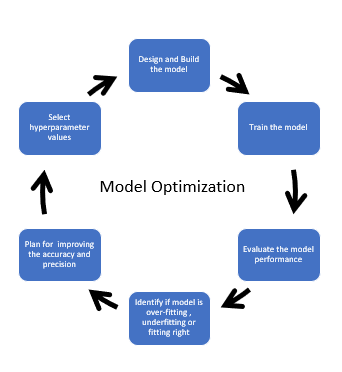可以使用以下技术执行超参数优化。 手动搜索 网格搜索:对指定超参数的所有可能组合进行穷举搜索，从而得到笛卡尔积。 随机搜索:超参数是随机选择的，不是每一个超参数的组合都被尝试。随着超参数数量的增加，随机搜索是一个更好的选择，因为它可以更快地得到超参数的良好组合。 贝叶斯优化:整合关于超参数的先验数据，包括模型的准确性或损失。先验信息有助于确定模型超参数选择的更好近似。 为了在TensorBoard中可视化模型的超参数并进行调优，我们将使用网格搜索技术，其中我们将使用一些超参数，如不同的节点数量，不同的优化器，或学习率等看看模型的准确性和损失。 为什么使用TensorBoard进行超参数优化? 一幅图片胜过千言万语，这也适用于复杂的深度学习模型。深度学习模型被认为是一个黑盒子，你发送一些输入数据，模型做一些复杂的计算，输出结果。 TensorBoard是Tensorflow的一个可视化工具包，用于显示不同的指标、参数和其他可视化，帮助调试、跟踪、微调、优化和共享深度学习实验结果 TensorBoard可以跟踪模型在每个训练迭代的精度和损失; 还有不同的超参数值。不同超参数值的跟踪精度将帮助您更快地微调模型。 我们将使用猫和狗数据集使用TensorBoard可视化标量、图形和分布。 https://www.kaggle.com/c/dogs-vs-cats/data 导入所需的库 导入TensorFlow和TensorBoard HParams插件以及Keras库来预处理图像和创建模型。 import tensorflow as tf from tensorboard.plugins.hparams import api as hp import datetime from tensorflow.keras.models import Sequential from tensorflow.keras.layers import Dense, Conv2D, Flatten, Dropout, MaxPooling2D from tensorflow.keras.preprocessing.image import ImageDataGenerator, img_to_array, load_img import numpy as np  加载TensorBoard notebook扩展 # Load the TensorBoard notebook extension %load_ext tensorboard  创建图像分类的深度学习模型 为训练设置关键参数 BASE_PATH = 'Data\\dogs-vs-cats\\train\\' TRAIN_PATH='Data\\dogs-vs-cats\\train_data\\' VAL_PATH='Data\\dogs-vs-cats\\validation_data\\'batch_size = 32 epochs = 5 IMG_HEIGHT = 150 IMG_WIDTH = 150  对训练图像进行缩放和不同的增强 train_image_generator = ImageDataGenerator( rescale=1./255, rotation_range=45, width_shift_range=.15, height_shift_range=.15, horizontal_flip=True, zoom_range=0.3)  重新调节验证数据 validation_image_generator = ImageDataGenerator(rescale=1./255)  为训练和验证生成成批的规范化数据 train_data_gen = train_image_generator.flow_from_directory( batch_size = batch_size, directory=TRAIN_PATH, shuffle=True, target_size=(IMG_HEIGHT, IMG_WIDTH), class_mode='categorical') val_data_gen = validation_image_generator.flow_from_directory(batch_size = batch_size, directory=VAL_PATH, target_size=(IMG_HEIGHT, IMG_WIDTH), class_mode='categorical')  为网格搜索（Grid Search）设置超参数 我们通过列出超参数的不同值或取值范围，使用了四个超参数来运行我们的实验。 对于离散超参数，将尝试所有可能的参数组合，对于实值参数，只使用下界和上界。 第一层的单元数量:256和512 dropout比例:范围在0.1到0.2之间。所以dropout比例是0。1和0。2。 优化器:adam, SGD, rmsprop 优化器的学习率:0.001,0.0001和0.0005， 我们还将准确率显示在TensorBoard 上 ## Create hyperparameters HP_NUM_UNITS=hp.HParam('num_units', hp.Discrete([ 256, 512])) HP_DROPOUT=hp.HParam('dropout', hp.RealInterval(0.1, 0.2)) HP_LEARNING_RATE= hp.HParam('learning_rate', hp.Discrete([0.001, 0.0005, 0.0001])) HP_OPTIMIZER=hp.HParam('optimizer', hp.Discrete(['adam', 'sgd', 'rmsprop']))METRIC_ACCURACY='accuracy'  创建和配置日志文件 log_dir ='\\logs\\fit\\' + datetime.datetime.now().strftime('%Y%m%d-%H%M%S') with tf.summary.create_file_writer(log_dir).as_default(): hp.hparams_config( hparams= [HP_NUM_UNITS, HP_DROPOUT, HP_OPTIMIZER, HP_LEARNING_RATE], metrics=[hp.Metric(METRIC_ACCURACY, display_name='Accuracy')], )  创建、编译和训练模型 超参数不是硬编码的，但从hparams字典为不同的参数:HP_DROPOUT , HP_NUM_UNITS ，HP_OPTIMIZER ，HP_LEARNING_RATE。 函数返回最后一个批次的验证准确性。 def create_model(hparams): model = Sequential([ Conv2D(64, 3, padding='same', activation='relu', input_shape=(IMG_HEIGHT, IMG_WIDTH ,3)), MaxPooling2D(), #setting the Drop out value based on HParam Dropout(hparams[HP_DROPOUT]), Conv2D(128, 3, padding='same', activation='relu'), MaxPooling2D(), Dropout(hparams[HP_DROPOUT]), Flatten(), Dense(hparams[HP_NUM_UNITS], activation='relu'), Dense(2, activation='softmax')]) #setting the optimizer and learning rate optimizer = hparams[HP_OPTIMIZER] learning_rate = hparams[HP_LEARNING_RATE] if optimizer == "adam": optimizer = tf.optimizers.Adam(learning_rate=learning_rate) elif optimizer == "sgd": optimizer = tf.optimizers.SGD(learning_rate=learning_rate) elif optimizer=='rmsprop': optimizer = tf.optimizers.RMSprop(learning_rate=learning_rate) else: raise ValueError("unexpected optimizer name: %r" % (optimizer_name,)) # Comiple the mode with the optimizer and learninf rate specified in hparams model.compile(optimizer=optimizer, loss='categorical_crossentropy', metrics=['accuracy']) #Fit the model history=model.fit_generator( train_data_gen, steps_per_epoch=1000, epochs=epochs, validation_data=val_data_gen, validation_steps=1000, callbacks=[ tf.keras.callbacks.TensorBoard(log_dir), # log metrics hp.KerasCallback(log_dir, hparams),# log hparams ]) return history.history['val_accuracy'][-1]  对于模型的每次运行，使用超参数和最终批次精度记录hparams都会被纪律。我们需要将最后一个批次的验证精度转换为标量值。 def run(run_dir, hparams): with tf.summary.create_file_writer(run_dir).as_default(): hp.hparams(hparams) # record the values used in this trial accuracy = create_model(hparams) #converting to tf scalar accuracy= tf.reshape(tf.convert_to_tensor(accuracy), []).numpy() tf.summary.scalar(METRIC_ACCURACY, accuracy, step=1)  用不同的超参数值运行模型 这里的实验使用网格搜索，并测试第一层单元数的所有可能的超参数组合，Dropout比例、优化器及其学习率，以及准确度用于准确性。 session_num = 0for num_units in HP_NUM_UNITS.domain.values: for dropout_rate in (HP_DROPOUT.domain.min_value, HP_DROPOUT.domain.max_value): for optimizer in HP_OPTIMIZER.domain.values: for learning_rate in HP_LEARNING_RATE.domain.values: hparams = { HP_NUM_UNITS: num_units, HP_DROPOUT: dropout_rate, HP_OPTIMIZER: optimizer, HP_LEARNING_RATE: learning_rate, } run_name = "run-%d" % session_num print('--- Starting trial: %s' % run_name) print({h.name: hparams[h] for h in hparams}) run('logs/hparam_tuning/' + run_name, hparams) session_num += 1  在HParams中可视化结果 python -m tensorboard.main --logdir="logs/hparam_tuning"  当按精度降序排序时，可以看到最优化的模型是256台，dropout比例为0.2,rmsprop优化器学习率为0.0005。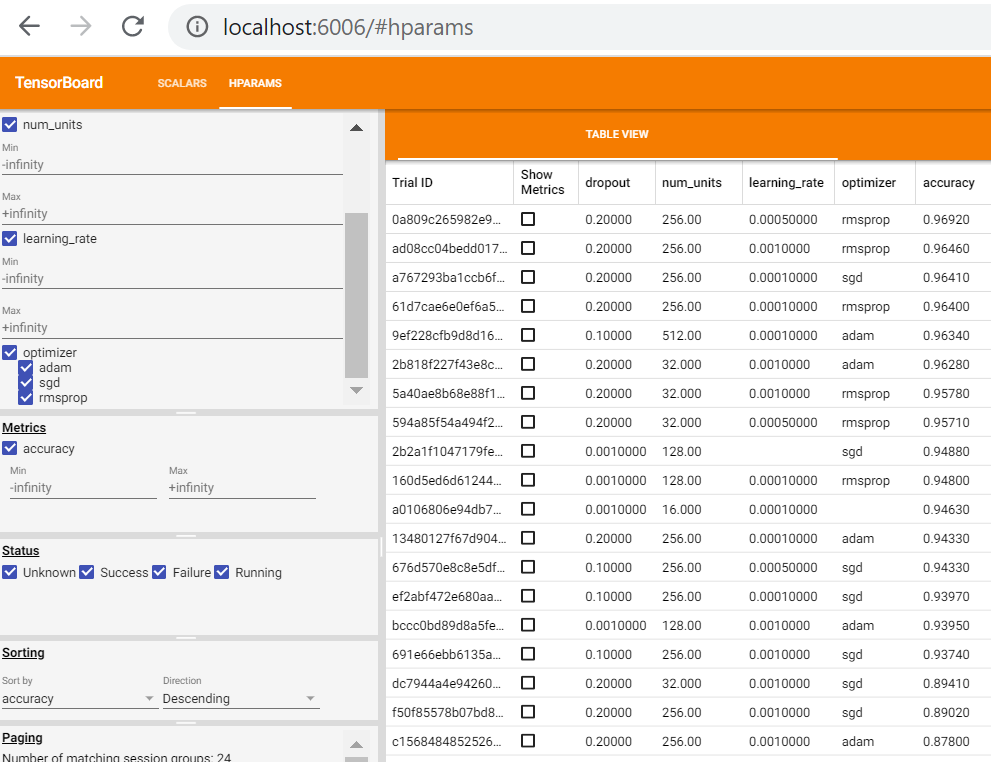在jupyter notebook中可以使用以下命令查看 %tensorboard --logdir='\logs\hparam_tuning'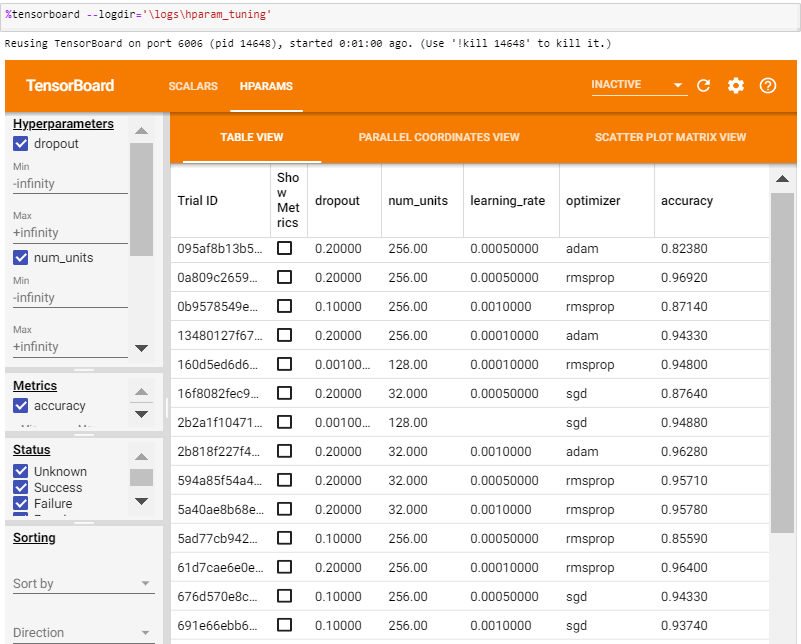在Tensorboard中使用Parallel Coordinates视图，显示每个超参数的单独运行情况并显示精度，查找最优化的超参数，以获得最佳的模型精度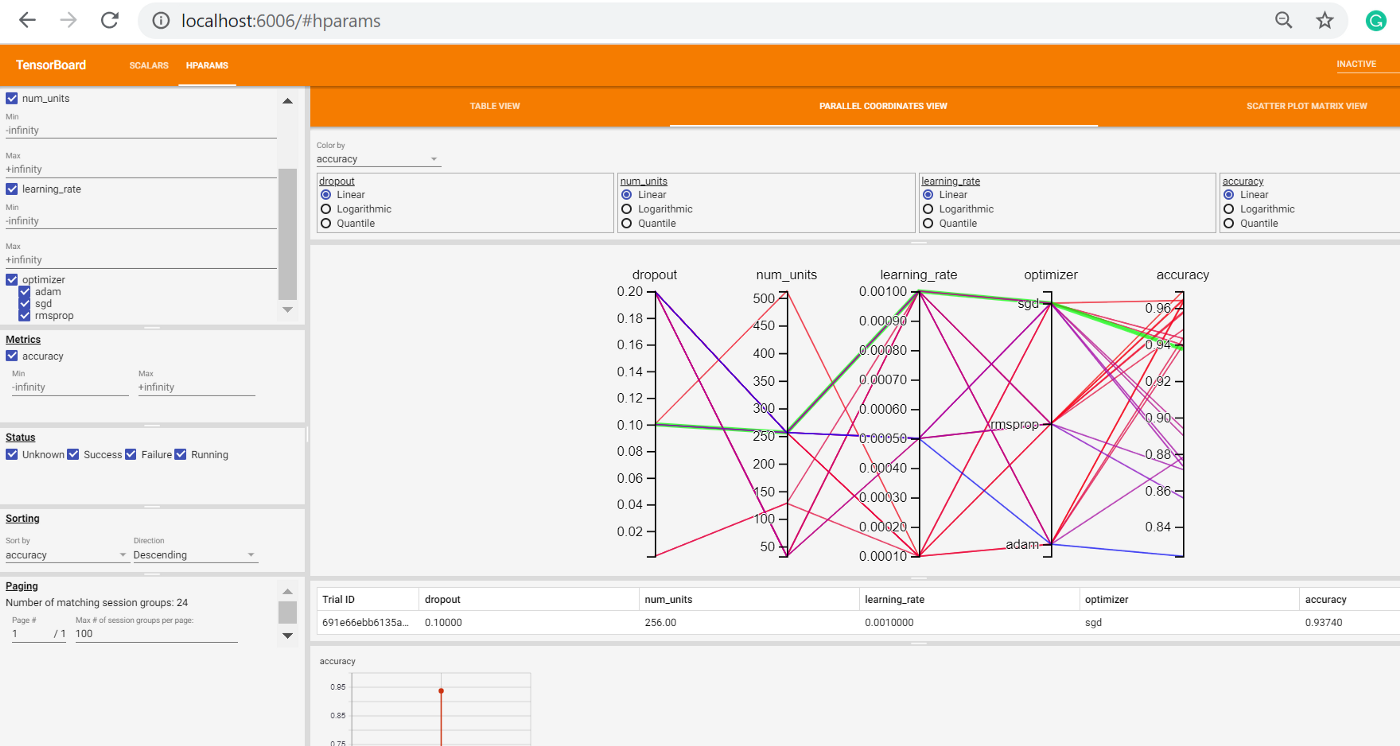总结 Tensorboard为超参数调优提供了一种可视化的方式来了解哪些超参数可以用于微调深度学习模型以获得最佳精度，更多的操作可以查看官方文档： https://www.tensorflow.org/tensorboard/hyperparameter_tuning_with_hparams 作者：Renu Khandelwal deephub翻译组  展开全文深度学习 神经网络 tensorflow • CFLAGS += -Wall -O2 -mcpu=cortex-a53 -mfloat-abi=softfp -mfpu=neon-vfpv4 -mno-unaligned-access -fno-...其中-Otime参数可能会报错，改为-Ofast，表示在-O2/-O3优化时，优先考虑执行速度进行优化 CFLAGS += -Wall -O2 -mcpu=cortex-a53 -mfloat-abi=softfp -mfpu=neon-vfpv4 -mno-unaligned-access -fno-aggressive-loop-optimizations -g -lmpi(INC_FLAGS) \$(LIBS)
-Wall：编译警告信息显示

-fno-aggressive-loop-optimizations：消除（或理解为“不允许”）程序中的无限循环行为

-mno-unaligned-access：进行对齐的memory访问

Hi3519A具有浮点运算单元和neon，文件系统中的库是采用软浮点和neon编译而成，因此所有板端代码编译时需要在Makefile里面添加选项-mcpu=cortex-a53、-mfloat-abi=softfp和-mfpu=neon-vfpv4，下面分别讲解这几个参数作用：

-mfloat-abi=softfp

ABI即“application binary interface”，即编译器将c代码编译成汇编代码时使用的一种规则
使用规范如下：
在编译带有浮点参数的函数时，有三种可能的编译选项：
-mfloat-abi=soft
-mfloat-abi=softfp
-mfloat-abi=hard
其中：
"soft"选项：表明不使用FPU硬件，而是使用GCC的整数算术运算来模拟浮点运算。
"softfp"选项：表明要使用FPU硬件来做浮点运算，只是，函数的参数传递到整数寄存器（r0-r3）中，然后再传递到FPU中。
"hard"选项：表明要使用FPU硬件来做浮点运算，并且，函数的参数直接传递到FPU的寄存器（s0、d0）中。

自动向量化选项
armcc编译器使用–vectorize选项来使能向量化编译，一般选择更高的优化等级如-O2或者-O3就能使能–vectorize选项。
gcc编译器的向量化选项-ftree-vectorize来使能向量化选项，使用-O3会自动使能-ftree-vectorize选项。

-mcpu=cortex-a53

它用于选择处理器类型
使用 -A或者–cpu Cortex-a53来指定指令集架构和CPU类型。
选项-mfpu=neon和-mcpu来指定cpu类型。如-mcpu=cortex-a5

-mfpu=neon-vfpv4

它用于选择NEON和VFP类型
gcc选择用-mfpu=vfpv3-fp16来指定为vfp协处理，而-mfpu=neon-vfpv4等就能指定为NEON+VFP结构。

总结：

选择浮点处理器和ABI接口类型
-mfloat-abi=soft：使用软件浮点库，不是用VFP或者NEON指令；
-mfloat-abi=softfp：使用软件浮点的调用规则，而可以使用VFP和NEON指令，编译的目标代码和软件浮点库链接使用；
-mfloat-abi=hard：使用VFP和NEON指令，并且改变ABI调用规则来产生更有效率的代码，如用vfp寄存器来进行浮点数据的参数传递，从而减少NEON寄存器和ARM寄存器的拷贝。

查看处理器是否支持NEON

#cat /proc/cpuinfo

看是否有如下内容，其中所用的cpu所支持的特性：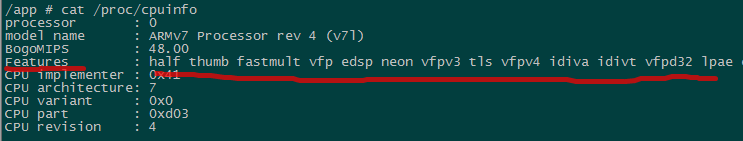其他参数：

参考：https://blog.csdn.net/rony2012/article/details/76614863

其中-Otime参数可能会报错，改为-Ofast，表示在-O2/-O3优化时，优先考虑执行速度进行优化


展开全文• Linux 下的库文件在命名时有一个约定,那就是应该以 lib 三个字母开头,由于所有的库文件都遵循 了同样的规范,因此在用-l 选项指定链接的库文件名时可以省去 lib 三个字母,也就是说 GCC 在对-lfoo 进行处理时,会自动去...gcc linux input integer
• 摘要 参数调优内容： 　1.... 　2.... 　3....　4. 其它相关参数 ...（1）innodb_buffer_pool_size .... 这个参数主要缓存innodb表的索引，数据，插入数据时的缓冲。为Innodb加速优化首要参数。   最重要的参数
• 此篇文章将简单讲解游戏中，场景的优化，以及制作规范，简单技巧和这样做的原因。 【前言】 优化是影响游戏体验的一个重要因素，好的优化，能够让玩家手感，体验达到最佳，而优化太差，会导致很多问题，比如内存...Unity 渲染
• ## 卷积神经网络(CNN)的参数优化方法

万次阅读 多人点赞 2016-07-22 10:11:00
著名： 本文是从 Michael Nielsen的电子书Neural Network and Deep Learning的深度学习那一章的卷积神经网络的参数优化方法的一些总结和摘录，并不是我自己的结论和做实验所得到的结果。我想Michael的实验结果更有...卷积神经网络 神经网络 深度学习
• ## 神经网络的参数优化方法

万次阅读 多人点赞 2018-10-23 14:05:42
著名： 本文是从 Michael Nielsen的电子书Neural Network and Deep Learning的深度学习那一章的卷积神经网络的参数优化方法的一些总结和摘录，并不是我自己的结论和做实验所得到的结果。我想Michael的实验结果更有...卷积神经网络 调参 神经网络设计 trick
• 自动机器学习超参数调整（贝叶斯优化）—介绍了例子 贝叶斯优化(Bayesian Optimization)深入理解 贝叶斯优化(BayesianOptimization) 简介 所谓优化，实际上就是一个求极值的过程，数据科学的很多时候就是求极值...
• 也许你可以将这个参数设置的更大，从而让你的超大的documdent可以写入es，但是es底层的lucene引擎还是有一个2gb的最大限制。 即使我们不考虑引擎层的限制，超大的document在实际生产环境中是很不好的。超大...性能
• ## 史上最强Tomcat8性能优化

万次阅读 多人点赞 2019-10-25 15:33:32
文章目录授人以鱼不如授人以渔目的服务器资源Tomcat配置优化Linux环境安装运行Tomcat8AJP连接执行器（线程池）3种运行模式部署测试用的web项目...测试报告调整Tomcat参数进行优化禁用AJP连接设置线程池最大线程数为150...Tomcat调优 Tomcat性能调优
• Spark性能优化：JVM参数调优 年轻代：主要是用来存放新生的对象。 老年代：主要存放应用程序中生命周期长的内存对象。 Spark中，垃圾回收调优的目标就是，只有真正长时间存活的对象，才能进入老年代，短时间存活的...spark
• 点击上方“码农进阶之路”，选择“设为星标”回复“面经”获取面试资料一、数据库设计方面1、对查询进行优化，应尽量避免全表扫描，首先应考虑在where及order by涉及的列上建立...
• #优化启动：非阻塞执行，牺牲CPU换取快速启动 CMD java -Djava.security.egd=file:/dev/./urandom -jar baye-eureka.jar 2、替换boot中的tomcat容器 &lt;!--web 模块--&gt; &lt;dependency&...
• 力争用数据给大家一个关于正则化，增加卷积层/全连接数，弃权技术，拓展训练集等参数优化方法的效果。 提高泛化能力的方法，一般来说，提高泛化能力的方法主要有以下几个： 正则化 增加神经网络层数 使用正确的代价...
• 3. 【强制】不要用 resultClass 当返回参数，即使所有类属性名与数据库字段一一对应，也需 要定义；反过来，每一个表也必然有一个与之对应。 说明：配置映射关系，使字段与 DO 类解耦，方便维护。  4. 【强制】...sql 索引 性能
• 说明：该笔记来自高性能可扩展MySQL数据库架构设计与优化，自行整理。 一、项目说明：该笔记的背景为电商平台项目，电商项目由于其高并发、多线程、高耗能等特性，在众多的项目类型中涉及的技术面最广，故以此为例...
• ## 华为C语言编程规范（精华总结）

万次阅读 多人点赞 2020-03-24 09:48:55
一般情况下，代码的可阅读性高于性能，只有确定性能是瓶颈时，才应该主动优化。 2、简洁为美 简洁就是易于理解并且易于实现。代码越长越难以看懂，也就越容易在修改时引入错误。写的代码越多，意味着出错的地方越多...
• 在各种类型的操作系统上的选择，在C语言编程角度，如何构建代码才能更好的指导编译器compiler进行优化，诸如数据对齐data alignment，数据类型data type的选择，C语言函数调用的参数传递方式，以及编译器对结构体和...c 编译器 cache
• C语言常规优化策略4 参数传递、宏定义、全局变量与汇编按照结构化程序设计的原则，一种语言，如果具有赋值、选择与循环三种结构，并严格按照这三种结构来组织程序，避免使用象goto语句这类使程序控制发生跳转的语言...语言 c 汇编 windows
• 2.我们在Controller类的Post方法的参数中加入@Valid注解，并使用BindingResult将错误信息作为日志打印到后台 @PostMapping public User create (@Valid @RequestBody User user, BindingResult errors){ ...spring
• ## 网络优化之net.ipv4.tcp_tw_recycle参数

万次阅读 多人点赞 2018-02-05 16:30:44
，但被拒绝了，其实，这对TCP规范，对TIME-WAIT来说，是利大于弊的。 那么问题来了 我们来看下，为什么这个状态能影响到一个处理大量连接的服务器，从下面三个方面来说： 新老连接（相同...
• 上节课我们主要介绍了深度神经网络的优化算法。包括对原始数据集进行分割，使用mini-batch gradient descent。然后介绍了指数加权平均（Exponentially weighted averages）的概念以及偏移校正（bias correction）...神经网络 深度学习 吴恩达 TensorFlow
• ## MySql优化

千次阅读 2019-01-18 09:50:19
什么是优化？ 合理安排资源、调整系统参数使MySQL运行更快、更节省资源。 优化是多方面的，包括查询、更新、服务器等。 原则：减少系统瓶颈，减少资源占用，增加系统的反应速度。 数据库性能参数 使用SHOW...sql
• 需求场景: 基于服务治理的原则，公司重构了很多项目，其中使用了dubbo作为服务框架。作为底层的服务存在，由上层的...代码 还有点乱，有还有优化的空间 dubboServiceFilter
• 尝试访问按照顺序的每一个path，如果可以有效的读区就直接向用户返回这个path对应的文件结束请求，否则继续向下访问，如果所有的path都找不到文件，就重定向到最后的参数uri上，因此，最后这个参数uri必须存在，而且...nginx...